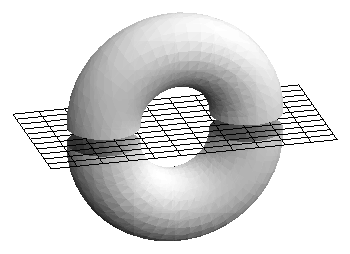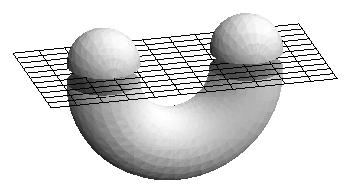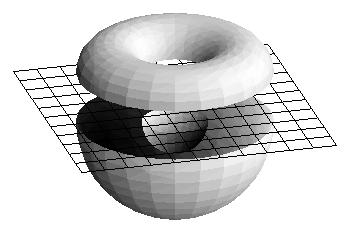The theory of tight submanifolds starts with attempts to generalize theorems about convex surfaces to topologically more complex surfaces such as the torus. For surfaces, it is possible to develop this generalization in terms of an elementary notion, the two-piece property, which then leads to the study of critical points of height functions and the theory of total absolute curvature. These notions can then be applied for higher-dimensional objects in higher-dimensional Euclidean spaces, producing a rich collection of examples and theorems in the global geometry of submanifolds.

An object in ordinary 3-dimensional space is said to have the two-piece property (TPP), if any plane cuts it into at most two pieces. Examples of surfaces with the TPP are spheres and ellipsoids and, more generally, the boundary of any bounded convex body. There are also non-convex objects with boundaries that have the TPP, for example a torus of revolution, or, more generally, a surface of revolution obtained by revolving a convex curve around an axis in the plane of the curve not meeting the curve.If we deform a sphere into a non-convex surface, for example a U-shaped object, or a sphere with a dent in it, the resulting surface will not have the TPP.For closed subsets, the TPP is equivalent to the condition that the intersection of the object with every closed half space is connected.

For compact surfaces (without boundary), the TPP is closely related to the study of critical points of height functions. Any plane in space can be considered a level set of a height function in a direction perpendicular to the plane. If a plane cuts a surface into more than two pieces, then a height function perpendicular to this plane must have at least one maximum or minimum on each piece. It follows that if a surface which does not have the TPP, there must be a height function with at least two (strict) local maxima on the surface. Conversely if a height function has two strict local maxima on a surface, then the half space above the level set containing the lower of the two will intersect the surface in at least two pieces. It follows that a surface has the TPP if and only if no height function restricted to the surface has more than on strict local maximum. A surface with this property is called tight. An equivalent definition for a surface to be tight is the condition that every local support plane be a global support plane.

The TPP is a topological condition so it applies to any surface in space, whether it be smooth, polyhedral, or just a one-to-one contiuous image of such a surface. If the surface happens to be sufficiently smooth, then it is possible to characterize the tightness condition in terms of its total or Gaussian curvature. Any point of positive curvature is a local extremum of the height function perpendicular to the tangent plane at the point. so the tightness condition implies that in any direction there is at most one point on the surface with positive curvature that is critical for that direction. For almost any direction, the maximum of the height function in that direction on the surface will occur at a point of positive curvature. It follow that if a smooth surface is tight, then the only strict local maxima of any height function must occur on the `outside', where the surface intersects its convex hull, the smallest convex set containing the surface.

For a smooth surface embedded in 3-dimensional space, tightness can be expressed in terms of the Gauss spherical image mapping, which sends each point of the surface to the point of the unit sphere centered at the origin with the same outer unit normal vector. (This definition assumes that a consistent field of unit normals has been chosen over the whole surface.). For any smooth surface without boundary, almost every point of the sphere is the image of at least one point with positive curvature, so the total area of the spherical image of the positive curvature part is at least 4 pi. For a tight smooth surface, almost every point of the sphere is the image of exactly one point of the surface with positive curvature, so the total area of the spherical image of the postive curvature part of the surface achieves the minimum value, namely 4 pi. Originally this property was used as the definition of tightness for smooth surfaces in ordinary space.

Although the first definition of tightness was given in terms of curvature, the critical point or TPP reformulation is much broader in scope. It applies not only to smooth surfaces in space but also to polyhedral surfaces. The critical point condition and the TPP extend naturally to surfaces embedded in higher-dimensional Euclidean spaces, and to immersions and to mappings with singularities.

It was Nicolaas Kuiper who made the first wide-ranging and systematic study of tight embeddings and immersions of surfaces, in three dimensions and higher. He produced tight embeddings of all orientable surfaces and tight immersions of all but three non-orientable surfaces in 3-dimensional space. He proved that two of the remaining surfaces, the real projective plane and the Klein bottle, could not be immersed tightly in 3-space even as topological surfaces and he conjectured that the final case, a real projective plane with one handle, could not be immersed tightly into 3-space. Only recently was Francois Haab able to prove that conjecture for smooth immersions, and even more recently Davide Cervone produced a surprising example to show that this surface can be immersed tightly as a polyhedral surface.

There are still a number of unsolved problems concerning immersions of surfaces into 4-space, but thanks to the work of Kuiper, the situation for five-space is better understood. First of all, Kuiper showed that any smooth immersion of a surface into Eucildean n-space for n >= 5 must lie in a 5-dimensional affine space, and moreover, if the image does not lie in a 4-dimensional subspace, then the surface is the real projective plane and the immersion is affinely equivalient to the Veronese embedding, an algebraic surface. An even stronger result by Kuiper and William Pohl states that any topological tight embedding of the real projective plane into 5-space, not lying in a 4-space, must be either the smooth Veronese embedding or a simplexwise linear embedding of a triangulation with exactly six vertices.

In order to appreciate the nature of the theorems of Kuiper, it is useful to consider the TPP and tightness for closed curves in Euclidean spaces. A convex curve in the plane has the TPP, whether it is smooth or polygonal or a more general topological embedding of the circle. If an embedded curve in the plane is not convex then there it does not coincide with the boundary of its convex hull, and there is a segment in the convex hull boundary containing points not in the curve. A line containing this segment bounds a half spece meeting the curve in at least two pieces, so a non-convex plane curve does not have the TPP. Furthermore, if a curve is not planar, then there are four points on the curve, in cyclic order, not lying in a plane, and it is possible to find a plane with the first and third on one side and the second and fourth on the other, thus separating the curve into at least four pieces. Thus a TPP curve in n-space is already contained in an affine 2-dimensional space.

In the case where the curve is smooth, we may recast the above result in terms of curvature to obtain a famous theorem in global differential geometry due to Werner Fenchel: total curvature of a smooth closed curve in any dimension is at least 2 pi, and if it is exactly 2 pi, the curve is convex.

For 2-dimensional surface, the TPP restricts not only the number of maxima and minima of height functions but also the total number of critical points. This follows from the critical point theorem of elementary Morse theory: for almost every height function on a smooth surface, the only critical points are maxima, minima, and ordinary saddle points, and the number of maxima plus the number of minima minus the number of saddles is constant, equal to the Euler characteristic of the surface, also described as the number of vertices minus the number of edges plus the number of triangles in any triangulation of the surface. By `integrating' this theorem over all height functions, we obtain one of the most famous of all theorems in global differential geometry, the Gauss-Bonnet Theorem, relating the integral of the total curvature of a smooth surface to its Euler characteristic. We may use this fact to obtain other characterizations of tightness.

For higher-dimensional manifolds, the situation is quite different. The two-piece property no longer serves to place such a strong restriction on the nature of the critical points of height functions. We say that an n-manifold is tight if the intersection of the object with any half-space is no more complicated than it has to be, i.e. the homology of the intersection is not greater than the homology of the whole object. For example, if a manifold is simply connected, we require that the intersection with every half space also be simply connected. Thus a closed hemisphere has the TPP but it is not tight since it is simply connected but there is a half space that intersects it in a circle.

Morse theory gives lower bounds for the numbers of critical points of various types for almost all smooth functions defined on a higher-dimensional manifold. Tightness for higher-dimensional submanifolds of a Euclidean space requires that almost all height functions have the minimal number of critical points. Fenchel's theorem was generalized by Chern and Lashof by considering the Lipschitz-Killing curvature of a submanifold of a higher-dimensional Euclidean space. The total measure of the absolute value of this curvature is equal to the integral over the sphere of the number of critical points of height functions on the submanifold. Fenchel's theorem is generalized by the result that an m-dimensional sphere is immersed with minimum total absolute curvature if and only if it is a convex hypersurface in an affine subspace of dimension m+1.

In this article, we will develop the theory of tight submanifolds primarily in the smooth and polyhedral situations. A related article based on the work of Nicolaas Kuiper will develop this theory for topological immersions.